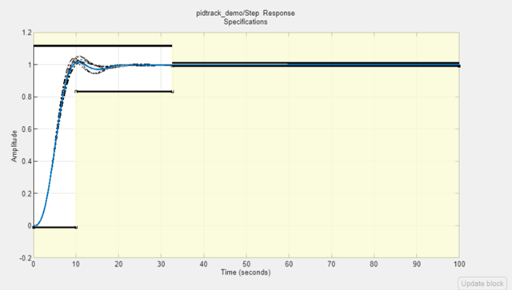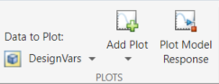Documentation

## Interact with Plots

This topic shows how to interact with plots in the Response Optimization tool.

### Response Plots

View model signals and the requirements applied to the signal using a response plot. You can also plot the frequency response of a system (requires Simulink® Control Design™ software).

The response plot shows the system response as it varies during optimization. You can also view the uncertain system responses in the plot.To plot a response plot:

1. In the Response Optimization tab of the tool, in the Data to Plot drop-down list, choose a signal, linear system, or requirement.2. Select a response plot in the Add Plot drop-down list.

You can add to an existing plot, or create a plot. The drop-down list has entries for the supported plot types for the given plot variable.

3. Display the current model response.

Click Plot Model Response. The current response appears as a thick line on the response plot.

4. Configure the plot display.

• To select the signal or systems to display, right-click within the white space in the plot, and choose from Signals and Systems in the menu.

• To control whether the response signal displays at intermediate steps during optimization, right-click within the white space in the plot and select Responses > Show Iteration Responses. The response at an intermediate step is based on parameter values at that intermediate step in the optimization.

• Modify plot properties.

You can change plot properties such as plot title, axis labels, axes limits, and units. Right-click the white space in a plot and select Axes Properties to open the Property Editor dialog box.

### Spider Plots

Compare the values of design variable sets or evaluated requirements using a spider plot.

Spider plots depict multivariate data using a separate axis for each variable. The various axes are arranged clockwise and have a common intersecting point.To plot a spider plot for a design variable set or evaluated requirements:

1. In the Response Optimization tab of the tool, in Data to Plot, choose design variables, or evaluated requirements.

2. Select a Spider plot in the Add Plot drop-down list.

You can add to an existing plot, or create a new one. The drop-down list has entries for the supported plot types for the given plot variable.

3. Configure the plot display.

To view only some of the variables or requirement values in a given plot, right-click the plot, and select the variables or requirements in the Show list.

For information on using a spider plot to compare design variables sets or evaluated requirements, see Compare Requirements and Design Variables Using Spider Plot

### Iteration Plots

Plot the values of design variables and requirements as they vary during optimization using an iteration plot.

Iteration plots depict the value of the plot variables for each iteration. The x-axis represents the iteration number.To plot an iteration plot for a design variable set or requirements:

1. In the Response Optimization tab of the tool, in Data to Plot, choose design variables or requirements.

2. Select an iteration plot in the Add Plot drop-down list.

You can add to an existing plot, or create a plot. The drop-down list has entries for the supported plot types for the given plot variable.

3. Configure the plot display.

To view scaled values of the plotted variables, right-click the plot, and select Show scaled values.

4. Save design variables and requirements values for a given iteration using the iteration plots.